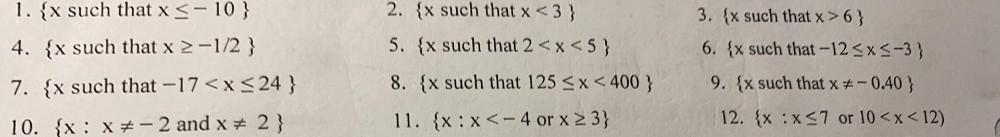Question:

# 1. {x such that x < -10} 2. x such that x <3} 5. x such that 2<x<5} 3. {x such that x>6) 6. {x such that -12<x<-3) 4. x such tha1. {x such that x < -10} 2. x such that x <3} 5. x such that 2<x<5} 3. {x such that x>6) 6. {x such that -12<x<-3) 4. x such that x 2-1/2 } 7. {x such that -17<x< 24} 8. x such that 125 < x < 400 } 9. {x such that x = -0.40 } 12. {x:x<7 or 10<x<12) 10. {x: X-2 and x + 2 } 11. {x:x<- 4 or x 23}